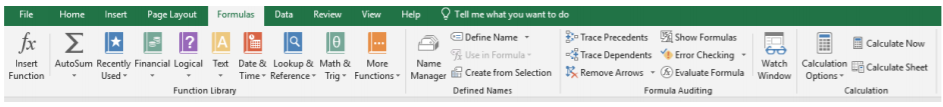# 18.1: Insert Function (or Introduction to Formulas in Excel)

$$\newcommand{\vecs}{\overset { \rightharpoonup} {\mathbf{#1}} }$$ $$\newcommand{\vecd}{\overset{-\!-\!\rightharpoonup}{\vphantom{a}\smash {#1}}}$$$$\newcommand{\id}{\mathrm{id}}$$ $$\newcommand{\Span}{\mathrm{span}}$$ $$\newcommand{\kernel}{\mathrm{null}\,}$$ $$\newcommand{\range}{\mathrm{range}\,}$$ $$\newcommand{\RealPart}{\mathrm{Re}}$$ $$\newcommand{\ImaginaryPart}{\mathrm{Im}}$$ $$\newcommand{\Argument}{\mathrm{Arg}}$$ $$\newcommand{\norm}{\| #1 \|}$$ $$\newcommand{\inner}{\langle #1, #2 \rangle}$$ $$\newcommand{\Span}{\mathrm{span}}$$ $$\newcommand{\id}{\mathrm{id}}$$ $$\newcommand{\Span}{\mathrm{span}}$$ $$\newcommand{\kernel}{\mathrm{null}\,}$$ $$\newcommand{\range}{\mathrm{range}\,}$$ $$\newcommand{\RealPart}{\mathrm{Re}}$$ $$\newcommand{\ImaginaryPart}{\mathrm{Im}}$$ $$\newcommand{\Argument}{\mathrm{Arg}}$$ $$\newcommand{\norm}{\| #1 \|}$$ $$\newcommand{\inner}{\langle #1, #2 \rangle}$$ $$\newcommand{\Span}{\mathrm{span}}$$$$\newcommand{\AA}{\unicode[.8,0]{x212B}}$$Since Excel is an object-oriented program (meaning that calculations must be completed in a physical location, before inserting a function, the user must select the cell they would like to add a formula to. For the purposes of this illustration, I will select the cell “C2”. When I select the Insert Function icon, an equals sign “=” is displayed in cell C2, and an Insert Function control panel is displayed.Using the Insert Function control panel, I can either navigate to the type of function I would like to use, or I can search for a function. For the purposes of this example, I would like to add the numbers “2” and “3” which are located in cell “A2” and “B2”. I search “add” because I want to add the two numbers together. Excel provides the “SUM” function as the option I think it wants. It also displays the formula that would be usedalong with a description of what it does. I agree with Excel that this is what I want to do, and click “OK”. Now a Function Arguments dialog box launches so I can correctly place the cells I would like to use in the equation.Because I had the cell selected (C2) where I wanted the sum of A2 and B2 to be, Excel automatically placed the cell range to be added together in the Number1 area. It also shares with me the numbers in those two fields and what the answer would be. If I was adding multiple cells in different areas together, I could use the Number2 and Number3 fields as well. When I begin to enter a cell in Number3, another field will be created (Number4). When I think my calculation and formula are correct, I press “OK”.Notice that the number “5” is correctly added in C2, and in the cell, the formula is shown in the formula bar.If I know the formula, I can also enter it manually via the keyboard into the formula bar as well. Remember to place a “=” sign first, and then the formula command, followed by the cells selected in brackets (). While this is a simple example, regardless of the complexity, formulas work the same.

This page titled 18.1: Insert Function (or Introduction to Formulas in Excel) is shared under a CC BY license and was authored, remixed, and/or curated by Nick Heisserer (Minnesota State Opendora) .